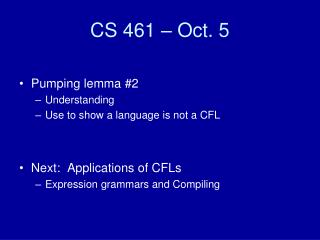# CS 461 – Oct. 5 - PowerPoint PPT PresentationDownload PresentationCS 461 – Oct. 5

CS 461 – Oct. 5
Download Presentation## CS 461 – Oct. 5

- - - - - - - - - - - - - - - - - - - - - - - - - - - E N D - - - - - - - - - - - - - - - - - - - - - - - - - - -
##### Presentation Transcript

1. CS 461 – Oct. 5 • Pumping lemma #2 • Understanding • Use to show a language is not a CFL • Next: Applications of CFLs • Expression grammars and Compiling

2. Pumping lemma L is a CFL implies: There is a p, such that for any string s, |s| >= p We can break up s into 5 parts uvxyz: | v y | >= 1 | v x y | <= p and uvixyiz  L. • How does this differ from the first pumping lemma?

3. In other words • If L is a CFL, then for practically any word w in L, we should be able to find 2 substrings within w located near each other that you can simultaneously pump in order to create more words in L. • Contrapositive: If w is a word in L, and we can always create a word not in L by simultaneously pumping any two substrings in w that are near each other, then L is not a CFL.

4. Gist • A string can grow in 2 places. • When we write recursive rules, we can add symbols to left or right of a variable. • Ex. S  #A# A  ε | 1A2 Look at derivation of: #111…222# • Even a complex rule like S  S1S1S2 can be “simplified”, and still only need 2 places to grow the string. • This is because any CFG can be written in such a way that there are no more than 2 symbols on the right side of .

5. Example • L = { 1n 2n } should satisfy the pumping lemma. Let p = 2. Strings in L of length >= 2 are: { 12, 1122, 111222, … }. All these words have both a 1 and a 2, which we can pump. Just make sure the 2 places to pump are within p symbols of each other. So let’s say they are in the middle. u v x y z 11111…1 1 ε 2 22222….2 Could p = 1 work?

6. Example 2 • { w # wR }. Think about where we can grow. Let p = 3. All strings of length 3 or more look like w # wR where | w | >= 1. As a technicality, would other values of p work?

7. Non-CFLs • It’s possible for a language to be non-CFL! Typically this will be a language that must simultaneously pump in 3+ places. • Ex. How could we design a PDA for { 1n2n3n }?

8. Adversary’s Revenge • In Game #1, how did we win? Ex. { 0n 1n }. • Adversary chose p. • We chose s. • Adversary broke up s = xyz subject to constraints. • We were always able to pump and find words outside L. • Game #2 strategy • Adversary’s constraints looser. The middle 3 parts have to be within p of each other. Can be anywhere in the string, not just in first p symbols of word.

9. { 1n 2n 3n } • Let p be any number. • Choose s = 1p2p3p. • Let s = uvxyz such that |vxy| <= p and |vy| >= 1. Where can v and y be? • All 1’s • All 2’s • All 3’s • Straddling 1’s and 2’s • Straddling 2’s and 3’s • In every case, can we find a word not in L?

10. More examples • { 1i 2j 3k : i < j < k } • What should we chose as s? • How can the word be split up? • { w w } • Let s = 0p1p0p1p. Where can v & y be? • Same section of 0’s and 1’s. • In neighboring sections • Either v or y straddles a border.